OR

# Learning About Fractions

## from SOITA Learning Technologies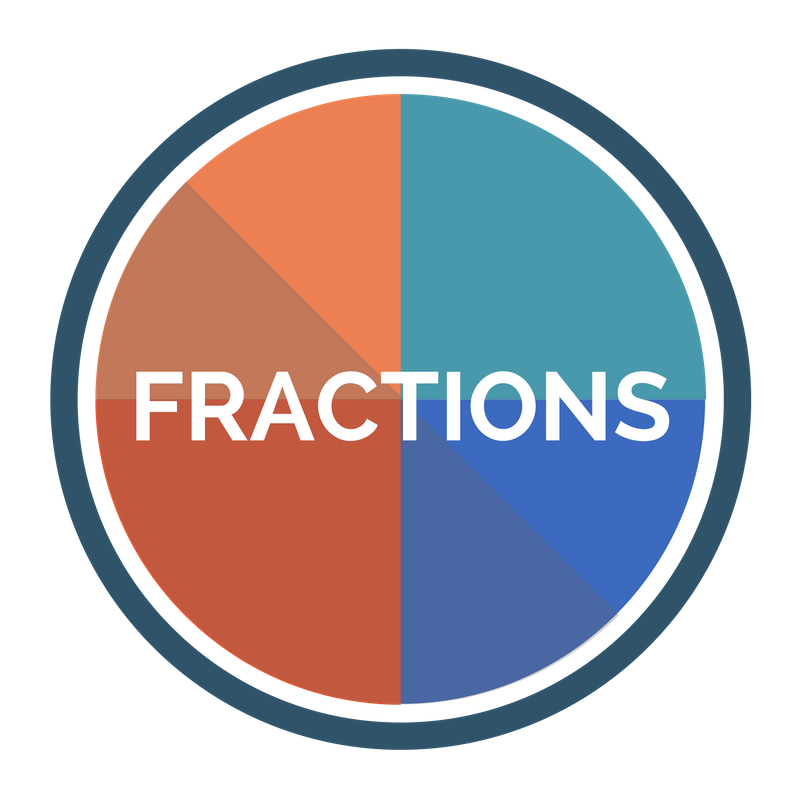Get the facts about fractions during this interactive videoconference. Students will identify fractions represented in a variety of ways, compare and order fractions with like denominators and recognize equivalent fractions.### Program Rating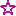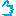based on 16 evaluation(s).

## About This Program

### Cost

Point to Point: \$150.00
Point to Point Premium: \$135.00
By Request: \$150.00
By Request Premium: \$135.00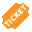This program is available as a point-to-point video conference at a pre-requested date and time at \$150 for CILC Members and \$135 for CILC Premium members.

### Length

45 minutes for Grades 3-4

### Target Audience

Education: Grade(s) 3, 4

No minimum

### Maximum participants:

Maximum of 30 students.

### Primary Disciplines

Mathematics, Technology/Information Science

### Program Delivery Mode

Videoconference - H.323 (Polycom, Cisco/Tandberg, LifeSize, etc...)
Zoom

## Booking Information

This program is available as a point-to-point video conference at a pre-requested date and time at \$150 for CILC Members and \$135 for CILC Premium members.

For more information contact CILC at (507) 388-3672

### Provider's Cancellation Policy

We will not charge for programs cancelled due to nature i.e. snow days. The full fee will be charged to sites which cancel with less than 48 hours notice.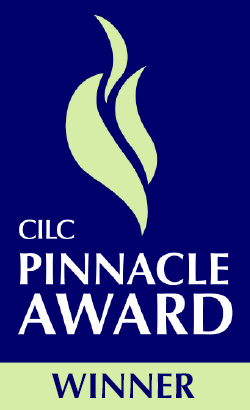Our mission at SOITA is to provide educators instructional technology services that will assist them in optimizing student learning and achievement. SOITA offers several choices for professional development and student learning via distance learning. Visit http://www.soita.org

Contact:
Kim Carter
kim@soita.org
9377466333

## Program Details

### Format

• Review equal parts
• Identify fractions as parts of whole
• Identify fractions as parts of a set
• Review fraction vocabulary, numerator, denominator
• Compare fractions with like denominators
• Put fractions with like denominators in order
• Equivalent fraction activity
• Identify equivalent fractions

### Objectives

• Identify fractions as part of a whole or part of a set
• Compare and order fractions with like denominators
• Identify equivalent fractions

### Standards Alignment

#### National Standards

CCSS.MATH.3.G.2 -- Partition shapes into parts with equal areas. Express the area of each part as a unit fraction of the whole. For example, partition a shape into 4 parts with equal area, and describe the area of each part as 1/4 of the area of the shape.
CCSS.MATH.3.NF.3 b. -- Recognize and generate simple equivalent fractions, e.g., 1/2 = 2/4, 4/6 = 2/3). Explain why the fractions are equivalent, e.g., by using a visual fraction model.
CCSS.MATH.4.NF.3 -- Understand a fraction a/b with a > 1 as a sum of fractions 1/b.

#### State Standards

Ohio State Standards

Grade 3
Math
MP.4 Model with mathematics.
3.NF.1 Understand a fraction 1/b as the quantity formed by 1 part when a whole is partitioned into
equal parts; understand a fraction a/b as the quantity formed by parts of size 1/b
3.NF.3 Explain equivalence of fractions in special cases and compare fractions by reasoning
about their size.
3.G.2 Partition shapes into parts with equal areas. Express the area of each part as a unit fraction
of the whole

Grade 4
Math
MP.2 Reason abstractly and quantitatively
MP.8 Look for and express regularity in repeated reasoning
4.NF.1 Explain why a fraction a/b is equivalent to a fraction (n × a)/(n × b) by using visual fraction
models, with attention to how the number and size of the parts differ even though the two
fractions themselves are the same size. Use this principle to recognize and generate
equivalent fractions.
4.NF.2 Compare two fractions with different numerators and different denominators, e.g., by
creating common denominators or numerators, or by comparing to a benchmark fraction
such as 1/2. Recognize that comparisons are valid only when the two fractions refer to the
same whole. Record the results of comparisons with symbols >, =, or <, and justify
conclusions, e.g., by using a fraction model.